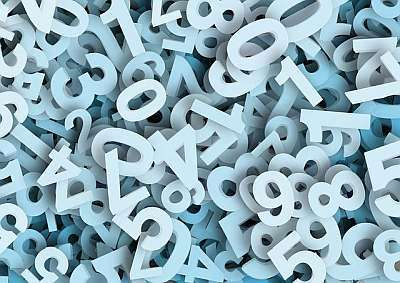# Decimal to Percent Calculator

Instructions: Use this calculator to convert a given decimal that you provide to a percent, showing all the steps. Please type in one decimal number (for example, a number like '3.4673' or a number like '.345279') in the form below:Type one decimal expression (Ex: 5.3445, or .4562)

## Using this Decimal to Percent Calculator

This decimal converter allows to conduct a very simple operation, but that can prove to be very useful. Indeed, decimals and percents are tightly related objects.

If I ask you "what is 0.40 converted into a percent", you probably can tell me directly, without thinking too much "40%". But if I asked you "what is 0.00000345 expressed as a percent", probably you would have to think how you would deal with that.

### How do you convert a decimal to percent

The strategy is simple. The base association is that the number 1 corresponds to 100%. Then, by direct proportionality, given a decimal $$D$$, all we need to do to convert it into a percent is to multiply it by 100.

So then, the decimal to percent formula for a decimal D is:

$P = D \times 100 \%$

So then, in the example of converting 0.00000345 expressed to a percent, we get $$P = 0.00000345 \cdot 100 \% = 0.000345 \%$$.

### Steps for the conversion of a decimal to percent

1. Identify the decimal you want to convert, and call it D
2. Make sure that the decimal D has the appropriate rounding, or no rounding whatsoever, according to your needs
3. Compute the percentage P, using the formula P = D * 100%
4. Don't forget to append the percentage sign "%" at the end of P### Examples: Converting decimals to percentages

What is 0.050 as a percentage?

Solution: According to our steps, first we identify the given decimal, which in this case is $$D = 0.050$$. The formula for expressing this decimal as a percentage is

$P = D \times 100 \% = 0.050 \times 100 \% = 5 \%$

Therefore, the conclusion is that 0.050 corresponds to 5%.

### Other calculators that relate decimals, fractions and percentages

Depending on what you are trying to achieve, it is likely that you will need some kind of conversion between decimals, fractions and percentages, and we have many calculators for you to do so.

It is very common to convert decimals to percents, especially when you trying to calculate something about finance, or related to interest rates. Sometimes interest rates are given in the common percentage format, but sometimes they are given as decimals, and you need to make the conversion.

Fractions, especially those that are less than one, can be commonly interpreted as percent in various contexts. For example, in the context of a mortgage, you may need to pay "an extra 1/4 of a point".

So then, you can use this fraction to percent calculator, but also depending on the context, you may have a better use for a fraction to decimal calculator.